# Check if Variable is equal to Multiple Values in JavaScriptLast updated: Jan 1, 2023
6 min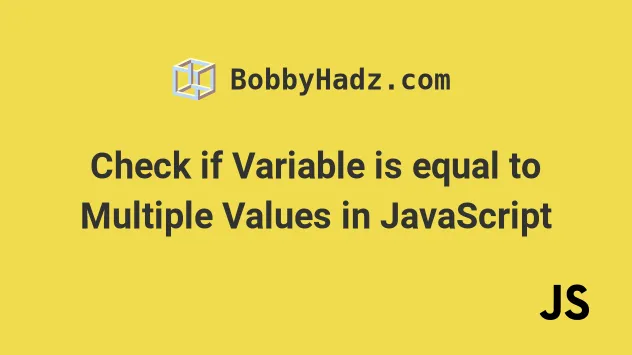## #Check if a Variable is equal to Multiple Values in JS

To check if a variable is equal to all of multiple values:

1. Wrap the values in an array and use the `every()` method to iterate over the array.
2. Check if each value is equal to the variable.
3. The `every` method will return `true` if the variable is equal to all of the values.
index.js
```Copied!```const str = 'hello world';

const val1 = 'hello world';
const val2 = 'hello world';
const val3 = 'hello world';

const result = [val1, val2, val3].every(value => {
return value === str;
});

console.log(result); // 👉️ true
``````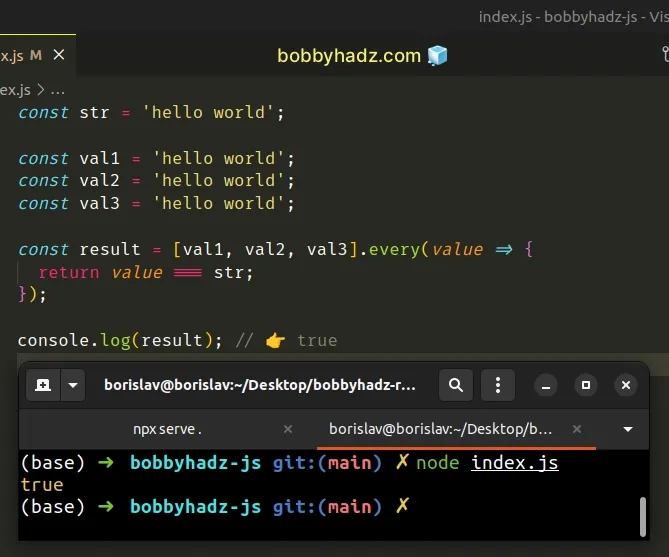The same approach can be used to compare multiple values.

index.js
```Copied!```const value1 = 10;
const value2 = 10;
const value3 = 10;

const arr = [value1, value2, value3];

const areEqual = arr.every(element => {
return element === arr;
});

console.log(areEqual); // 👉️ true
``````

The function we passed to the Array.every method gets called with each element in the array until it returns a falsy value or iterates over the entire array.

We compare each value against the variable and return the result.

If the comparison fails, the `every()` method short-circuits and returns `false`, otherwise, it returns `true`.

## #Check if a variable is equal to all of multiple values using && operator

An alternative approach is to use the logical AND (&&) operator.

If all comparisons return `true`, all values are equal to the variable.

index.js
```Copied!```const str = 'hello world';

const val1 = 'hello world';
const val2 = 'hello world';
const val3 = 'hello world';

if (str === val1 && str === val2 && str === val3) {
// 👇️ this runs
console.log('✅ variable is equal to all of the values');
} else {
console.log('⛔️ variable is not equal to all of the values');
}
``````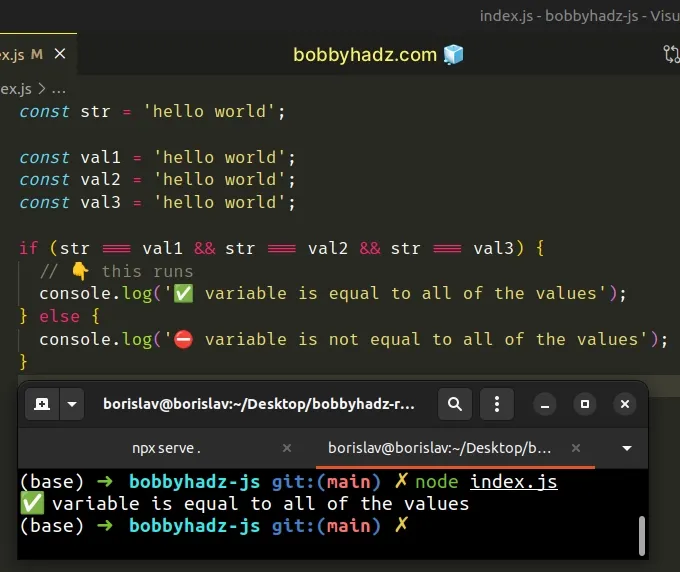We used the logical AND (&&) operator to chain multiple equality checks.

The logical AND (&&) operator returns the value to the left if it's falsy, otherwise, it returns the value to the right.

All the equality checks have to return `true` for the `if` block to run.

## #Check if a Variable is equal to One of Multiple Values in JS

To check if a variable is equal to one of multiple values:

1. Wrap the values in an array.
2. Call the `includes()` method on the array.
3. The `includes` method will return `true` if the value is contained in the array.
index.js
```Copied!```const str = 'hello world';

const val1 = 'hello world';
const val2 = 'bye world';
const val3 = 'test world';

if ([val1, val2, val3].includes(str)) {
console.log(
'✅ variable is equal to at least 1 of the values',
);
} else {
console.log('⛔️ variable is not equal to any of the values');
}
``````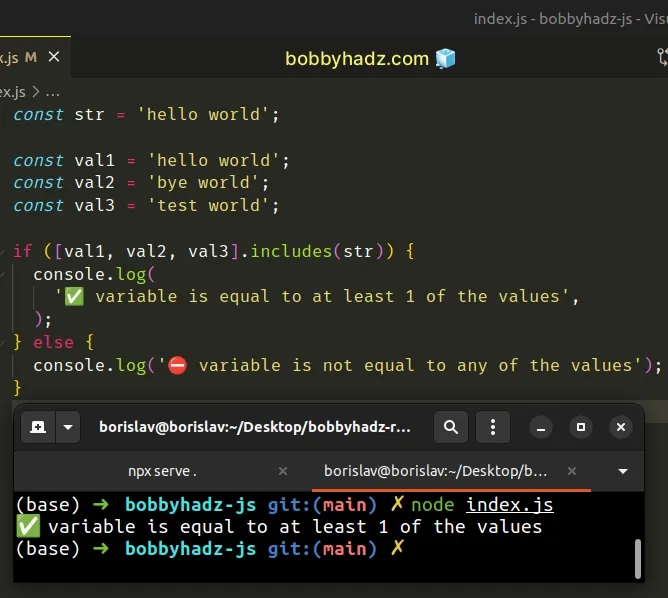We used the Array.includes() method to check if a value is contained in an array of values.

The method returns `true` if the value is contained in the array and `false` otherwise.

## #Checking if three values are equal using a `Set` object

If you need to check if the three values are equal, you can also pass the values to the Set() constructor.

`Set` objects only store unique values, so any duplicates passed to the constructor get automatically removed.

index.js
```Copied!```const set1 = new Set(['a', 'a', 'a']);
console.log(set1); // 👉️ {'a'}
``````

We can pass the values to the `Set()` constructor and if the size of the `Set` is equal to `1`, then all of the passed-in values are equal.

index.js
```Copied!```const val1 = 'apple';
const val2 = 'apple';
const val3 = 'apple';

if (new Set([val1, val2, val3]).size === 1) {
console.log('✅ The 3 values are equal');
} else {
console.log('⛔️ The values are NOT equal');
}
``````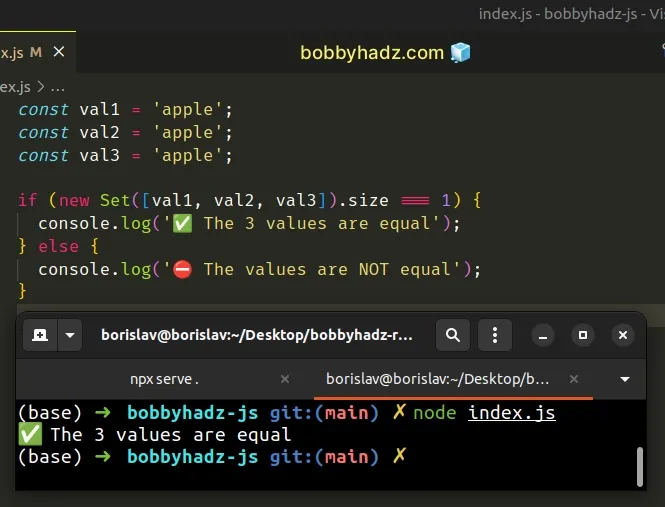The `Set` object doesn't contain duplicate values, so all of the duplicates got removed when initializing the `Set`.

If the new `Set` contains a single element, then all of the passed-in values are equal.

## #Check if a Variable is equal to One of Multiple Values using `indexOf`

This is a three-step process:

1. Wrap the values in an array.
2. Call the `indexOf()` method on the array.
3. If the method doesn't return `-1`, the variable is equal to one of the values.
index.js
```Copied!```const str = 'hello world';

const val1 = 'hello world';
const val2 = 'bye world';
const val3 = 'test world';

if ([val1, val2, val3].indexOf(str) !== -1) {
// 👇️ this runs
console.log('✅ variable is equal to at least 1 of the values');
} else {
console.log('⛔️ variable is not equal to any of the values');
}
``````

We used the Array.indexOf method to check if a value is contained in an array of values.

The method returns the index of the first occurrence of the value in the array or `-1` if the value is not contained in the array.

The `if` statement checks if the return value of the `indexOf` method is not equal to `-1`.

If the condition is met, the variable is equal to at least 1 of the values.

## #Check if Variable Doesn't Equal Multiple Values in JavaScript

To check if a variable is not equal to multiple values:

1. Check if the variable is not equal to the values using the strict inequality (!==) operator.
2. Use the logical AND (&&) operator to chain multiple conditions.
3. If all conditions pass, the variable is not equal to any of the values.
index.js
```Copied!```const a = 'one';
const b = 'two';
const c = 'three';

if (a !== b && a !== c) {
// 👇️ this runs
console.log('✅ a is not equal to b and c');
} else {
console.log('⛔️ a is equal to b or c, or both');
}

// ✅ Check if variable is not equal to multiple values (every())
const notEqual = [b, c].every(value => value !== a);
console.log(notEqual); // 👉️ true
``````

We used the strict inequality (!==) operator to check if the variable `a` is not equal to the variables `b` and `c`.

The operator returns a boolean result:

• `true` if the values are not equal
• `false` if the values are equal

We used the logical AND (&&) operator to chain the two conditions.

The `if` block will only run if both conditions are met.

The `&&` operator is evaluated from left to right. If the first condition in our `if` statement returns `false`, the operator short-circuits and doesn't evaluate the second condition.

If the `a !== b` part of the condition returns `false`, the `a !== c` part is not evaluated at all.

For example, in the following `if` statement, the `10 > 1` condition is never evaluated.

index.js
```Copied!```if (5 > 50 && 10 > 1) {
}
``````

We first check if `5 > 50` and get a value of `false`, so the `&&` operator short-circuits.

You can also use the `Array.includes()` method to check if a variable does not equal multiple values.

## #Check if Variable Doesn't Equal Multiple Values using `includes()`

This is a three-step process:

1. Wrap the values in an array.
2. Use the `Array.includes()` method to check if the variable is not contained in the array.
3. The method will return `true` if the variable doesn't equal any of the values.
index.js
```Copied!```const a = 'one';
const b = 'two';
const c = 'three';

const notEqual = ![b, c].includes(a);
console.log(notEqual); // 👉️ true

if (a !== b && a !== c) {
// 👇️ this runs
console.log('a is not equal to b and c');
} else {
console.log('a is equal to b or c, or both');
}
``````

We wrapped the `b` and `c` variables into an array and used the `Array.includes()` method to check if the `a` variable is contained in the array.

The `Array.includes()` method takes a value and returns `true` if the value is contained in the array and `false` otherwise.

index.js
```Copied!```console.log([b, c].includes(a)); // 👉️ false
console.log(![b, c].includes(a)); // 👉️ true
``````

We used the logical NOT (!) operator to negate the call to the `includes()` method.

When used with boolean values, like in the example, the operator flips the value, e.g. `true` becomes `false` and vice versa.

index.js
```Copied!```console.log(!true); // 👉️ false
console.log(!false); // 👉️ true
``````

The expression returns `true` if the variable doesn't equal the values and `false` otherwise.

You can also use the `Array.every()` method to check if a variable does not equal multiple values.

## #Check if Variable Doesn't Equal Multiple Values using `every()`

This is a three-step process:

1. Wrap the values in an array.
2. Use the `Array.every()` method to iterate over the array.
3. Check if each value is not equal to the variable and return the result.
index.js
```Copied!```const a = 'one';
const b = 'two';
const c = 'three';

const notEqual = [b, c].every(value => value !== a);
console.log(notEqual); // 👉️ true

if (a !== b && a !== c) {
// 👇️ this runs
console.log('a is not equal to b and c');
} else {
console.log('a is equal to b or c, or both');
}
``````

We wrapped the `b` and `c` variables into an array.

The function we passed to the `Array.every()` method gets called with each element in the array.

On each iteration, we check if the current value is not equal to the `a` variable and return the result.

The `every()` method checks if the condition is met for all array elements.

If it is, the method returns `true`, otherwise, `false` is returned.

I wrote a book in which I share everything I know about how to become a better, more efficient programmer.You can use the search field on my Home Page to filter through all of my articles.